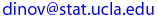Ivo Dinov
UCLA Statistics, Neurology, LONI
 Courses SOCR Ivo Dinov's Home SiteMap Software Contact

Multidimensional Normal (Gaussian) Distribution

Centralized (unnormalized) multidimensional Normal (Gaussian) distribution is denoted by: N(0, K) where the mean is 0 (zero) and the covariance matrix is K = ( Ki,j ) = Cov(Xi, Xj) .  K is symmetric by the identity, i.e.,  Cov(Xi, Xj) =  Cov(Xj , Xi) . Let  X = [X1, X2, X3, ...Xn]T  and  n-dimensional derivatives are written as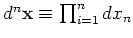.

The density function of N(0, K) is exp ( -1/2  XT K-1 X ), normalizing constant of the N(0, K) density is: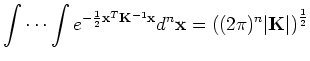(1)

where |K| = det( K ) is the determinant. In general, if multidimensional Gaussian is not centered then the (possibly offset) density has the form:  exp [ -1/2  (X-μ)T K-1 (X-μ) ].

• Because K-1  is real and symmetric (since (K-1)T =  (KT)-1 ), if  AK  we can decompose  A  into A  =  T Λ T-1, where T is an orthonormal matrix ( TT T =  I ) of the eigenvectors of  A and  Λ is a diagonal matrix of the eigenvalues of  A. Then the multidimensional Gaussian density can be expressed as: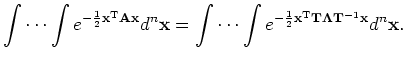(2)

However, T is orthonormal and we have T-1 = TT . Now define a new vector variable Y = TT X, and substitute in (2):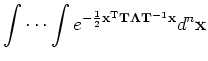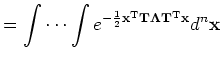(3)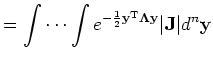(4)

where  | J | is the determinant of the Jacobian matrix J = ( Jm,n ) = ( ∂Xm / ∂Yn). As X = (TT)-1Y = Y ,  J T and thus | J | = 1.

• Because Λ  is diagonal, the integral (4) may be separated into the product of n independent Gaussians, each of which we can integrate separately using the 1D Gaussian: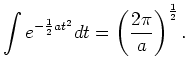(5)

Summarizing: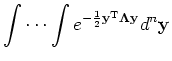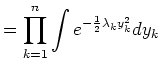(6)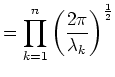(7)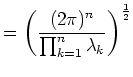(8)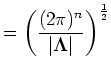(9)

Orthonormal matrix multiplication does not change the determinant, so we have

| A | = | T Λ T-1| = | T | | Λ | | T-1| =  | Λ |

And therefore: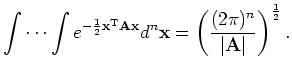(10)

Substituting back in for K-1  ( AK and | K-1 | = 1 / |K| ), we get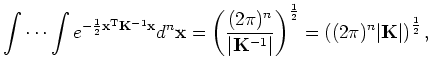(11)

as we expected.

 Ivo Dinov's Home http://www.stat.ucla.edu/~dinov Visitor number, since Jan. 01, 2002 © I.D.Dinov 1997-2007
Last modified on GMT by